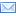#Difference Between Similar Terms and Objects

# Difference Between Average and MedianAverage vs Median

Median is the number representing the Ã«middleÃ­ of a given data sample which has been arranged numerically, given the sample of numbers is odd when counted. For a data set that is even in number when counted, the median in this case is got by dividing the sum of the two middle numbers by 2. Statistics and probability theory describes the median as the numeric value that divides a sample into two equal halves, that is a lower half and a higher half. Data set includes a sample and a population among others.

In any sample, the higher half will at most consist of values greater than the median while the lower half will consist of values less than the median at most. If both Ã«halvesÃ­ have less than half the sample, then that will mean that part of the sample will be exactly equal to the median. Take for instance, if x < z, then y will be the median of the sample x, y, z and if x < z

Median may at times be used to represent the measure of location where a distribution is oblique, when the last values are not known or when outside values are not important, if for instance they may result in measurement errors. Theoretically, median is very difficult to handle and this is a major disadvantage.

Average is commonly (and mistakenly) referred to as mean but average does not always equal to mean. The Ã«commonÃ­ average is the one referred to as the mean but definitely, average does not equate to mean.
From a practical point of view, there is just a marginal difference between median and mean for a given sample, theoretically, the differences may be stark. One major fact is that the difference between the two cannot easily be understood and is a common source of confusion for many people. Thus, the word Ã«averageÃ­ is often used when in actual sense the information was meant to represent mode or median.

Summary
Median is the middle number of a given dataset (if itÃ­s odd) while average is a definition of arithmetic mean, which is the most oft-cited average.
The value of median depends on the number of elements in a data set (odd or even) while average does not.

### Search DifferenceBetween.net :

Custom Search

Help us improve. Rate this post!(7 votes, average: 3.57 out of 5)Loading...Email This Post : If you like this article or our site. Please spread the word. Share it with your friends/family.

### 1 Comment

1. very useful information

Please note: comment moderation is enabled and may delay your comment. There is no need to resubmit your comment.

Articles on DifferenceBetween.net are general information, and are not intended to substitute for professional advice. The information is "AS IS", "WITH ALL FAULTS". User assumes all risk of use, damage, or injury. You agree that we have no liability for any damages.